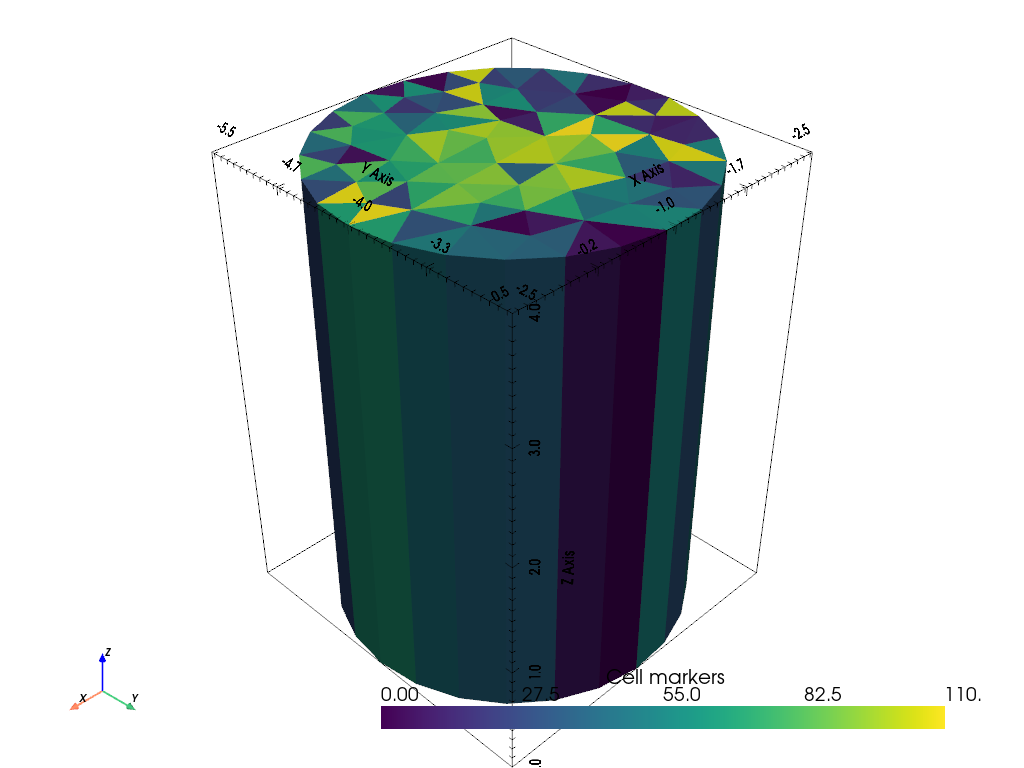# Extrude a 2D mesh to 3D#

This example shows how to extrude a 2D mesh to 3D. This can be helpful for closed laboratory geometries for example. If you are looking for more flexible ways to create 3D meshes, have a look at TetGen and Gmsh.

```import numpy as np

import pygimli as pg
import pygimli.meshtools as mt
```

We start by generating a 2D mesh.

```plc = mt.createCircle([-1, -4], radius=1.5, area=0.1, nSegments=25)
circle = mt.createMesh(plc)
for cell in circle.cells():
cell.setMarker(cell.id())
ax, cb = pg.show(circle, circle.cellMarkers(), label="Cell Markers")
```We now extrude this mesh to 3D given a z vector.

```z = np.geomspace(1, 5, 5)-1
cylinder = pg.meshtools.extrudeMesh(circle, a=z)
pl, _ = pg.show(cylinder, cylinder.cellMarkers(), label="Cell markers")
pl.show()
``````False
```

Gallery generated by Sphinx-Gallery